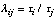# Manual Page for Command >>> TAU

Syntax
>>> TAU: (-)niter

Parent Command
>> ERROR

Subcommand
-

Description
If multiple observation types (e.g., pressure, flow rate, prior information, etc.) are used to estimate model parameters, the relative weights assigned to each type is given by the ratiowhere taui are scalars such that Ci = taui * Vi . Here, C is the covariance matrix of all observations of type i (a submatrix of covariance matrix Cii), and Vi is a positive symmetric matrix. By default, tau is fixed at 1. This command allows lambda to be updated in an iterative process, where tau is recalculated every multiple of niter iterations and is given by:If a negative number is provided for niter, prior information is excluded from the process. Recall that taui refers to all data of a certain observation type (i.e., not to individual data sets). The process assigns weights such that the relative contribution of each observation type to the objective function tends to 1/omega, where omega is the number of observation types used in the inversion. It should also be realized that the Fisher model test becomes meaningless if lambda is updated during the inversion because the test will always be fulfilled by definition. While this option provides flexibility in reassigning relative weights to data of different types, it is instead suggested to carefully select the standard deviations of the observations and prior parameter estimates (see command >>>> DEVIATION (p/o)) based on potential measurement and modeling errors.

Example
> COMPUTATION
>> ERROR
>>> update TAU every : -3 rd iteration
<<<